# Physics: Building Blocks Flashcards Preview

## MCAT > Physics: Building Blocks > Flashcards

Flashcards in Physics: Building Blocks Deck (28):
1

## To decrease a value by the percentages listed below, you would Multiply Original by _______ (in decimal and in fraction): 20%, 25%, 33%, 50%, 67%, 75%, 80%, 100%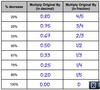2

## To raise a value by the percentages listed below, you would Multiply Original by _______ (in decimal and in fraction): 20%, 25%, 33%, 50%, 67%, 75%, 80%, 100%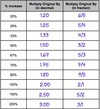3

4

5

6

7

## What are the fractions for Sin(θ) and Cos(θ)? For (θ) = 0°, 30°, 45°, 60°, 90°, 180°, 270°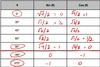8

## What are the areas and volume formulas for spheres and cylinders?9

## What are the areas and volume formulas for triangles and rectangles and squares?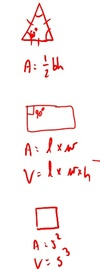10

## Define y= log [X] and y=ln (X)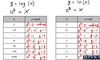11

## Given that PV = nRT, if the pressure is held constant which graph represents the relationship between temperature and volume?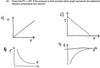12

## Given that PV = nRT, if the pressure is held constant what graph represents the relationship between temperature and volume?13

## Given that PE = -Gmm/r, which of the following graphs represents the relationship between PE and r?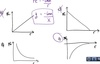14

## Which of the following graphs demonstrates an object in free fall? (Assume g = 10 m/s2)15

## How would a graph demonstrate an object moving at a constant positive velocity? x-axis: t y-axis: d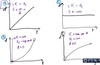16

17

## What graph correctly depicts v vs t for an object dropped from rest? (Assume g = 10 m/s2) x-axis: t y-axis: v

### Slope = acceleration18

## Which of the following graphs correctly depicts vy vs t for a projectile thrown vertically upward? (Assume g = 10 m/s2) x=v y=t19

## Kinematics Equations20

## A rock is dropped from a cliff that is 100 m above ground level. How long does it take the rock to reach the ground? (Note: Use g = 10 m/s2) A) 4.5 s B) 10 s C) 14 s D) 20 s

### Equation: XAT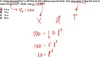21

## Starting from a resting position, a certain toy catapult applies a constant force of 3.0 N to a projectile with a mass of 0.06 kg. How long will it take for the projectile to move 1.0 m? A) 0.02 s B) 0.04 s C) 0.20 s D) 0.40 s

### Equation: TAX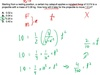22

## In the absence of air resistance, what maximum height will be reached by a 5-kg projectile with an initial vertical speed of 50 m/s? (Note: Use g =10 m/s2.) A) 25 m B) 50 m C) 125 m D) 250 m23

## Suppose that a ball is thrown vertically upward from earth with velocity v, and returns to its original height in a time t. If the value of g were reduced to g/6 (as on the moon), then t would: A) increase by a factor of 6. B) increase by a factor of 61/2 C) decrease by a factor of 6. D) decrease by a factor of 61/2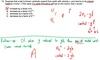24

## Two identical projectiles are given the same horizontal speed, but one is launched horizontally while the other is launched at an angle of 10° above the horizontal. If the projectiles do NOT experience air resistance, how will their time spent in flight and horizontal distance traveled compare? A) The horizontal projectile will travel farther and be in the air longer. B)The horizontal projectile will travel the same distance but will be in the air a shorter amount of time. C) The horizontal projectile will travel less distance but will be in the air longer. D) The horizontal projectile will travel less distance and be in the air a shorter amount of time.25

## Two projectiles are propelled with the same initial vertical speed but with different initial horizontal speeds. If air resistance is negligible, how will their time in the air and horizontal flight distance compare? A) They will remain in the air different amounts of time but travel the same distance. B) They will remain in the air different amounts of time and travel different distances. C) They will remain in the air the same amount of time and travel the same distance. D) They will remain in the air the same amount of time but travel different distances.26

27

28